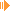Rasterising Lines, Circles and Ellipes - Bresenham AlgorithmsDownload Applet with Source (42kb)Download my Article "Notes About Rasterizing Lines And Circles" (570kb)

This applet demonstrates how to rasterise lines, circles and ellipse applying different algorithms - bad and slow ones, but also nice and faster ones. I will not describe them in detail, look at the links below and investigate the source code - then you will learn the most and see the differences between the various approaches.

• Line Raster Algorithms
• Digital Differential Analyzer Algorithm: the simple apporach - evaluates the standard line equation using the slope.
• Bresenham Algorithm using floating point numbers
• Bresenham Algorithm using integer numbers
• Circle Raster Algorithms:
• Simple approach 1: evaluates the circle equation and make use of 2-way symmetry... and gives really bad resutls
• Simple approach 2: evaluates the circle equation and make use of 2-way symmetry... giving good results
• Bresenham Algorithm using floating point numbers
• Bresenham Algorithm using integer numbers
• Ellipse Raster Algorithms:
• Bresenham Algorithm using integer numbers

Of course, I didn't re-invented the wheel, but I coded the line and circle algorithms by myself. But I have to admit that I wanted to add the ellipse functionality for completeness and I borrowed the implementation from  - by the way an awesome article.

References:

Sunshine, November 2k10

This site is part of Sunshine's Homepage